• 原理：利用复化梯形公式，复化Simpson公式，计算积分。步骤：import math"""测试函数"""def f(x,i):if i == 1:return (4 - (math.sin(x)) ** 2) ** 0.5if i == 2:if x == 0:return 1else:return math.sin(x) / xif i...

原理：

利用复化梯形公式，复化Simpson公式，计算积分。

步骤：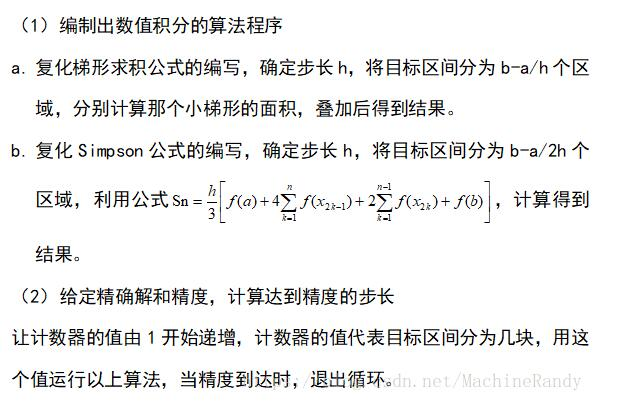import math

"""测试函数"""

def f(x,i):

if i == 1:

return (4 - (math.sin(x)) ** 2) ** 0.5

if i == 2:

if x == 0:

return 1

else:

return math.sin(x) / x

if i == 3:

return (math.exp(x)) / (4 + x ** 2)

if i == 4:

return math.log(1+x,math.e) / (1 + x ** 2)

"""打印显示函数"""

def p(i,n):

return "第" + str(i) + "题,n=" + str(n) + "时的积分值为："

"""复化Simpson函数"""

def Simpson(a, b, n, i):

h = (b - a) / (2 * n)

F0 = f(a,i) + f(b,i)

F1 = 0

F2 = 0

for j in range(1,2 * n):

x = a + (j * h)

if j % 2 == 0:

F2 = F2 + f(x,i)

else:

F1 = F1 + f(x,i)

SN = (h * (F0 + 2 * F2 + 4 * F1)) / 3

print("复化Simpson函数" + p(i,n) + str("%-10.7f"%(SN)))

return SN

def T(a, b, n, i):

h = (b - a) / n

F0 = f(a,i) + f(b,i)

F = 0

for j in range(1,n):

x = a + (j * h)

F = F + f(x,i)

SN = (h * (F0 + 2 * F)) / 2

print("复化梯形函数" + p(i,n) + str("%-10.7f"%(SN)))

return SN

def SimpsonTimes(x):

n = 1

y = Simpson(0, math.pi/4, n, 1)

while(abs(y - 1.5343916) > x):

n = n + 1

y = Simpson(0, math.pi/4, n, 1)

else:

return n

def Times(x):

n = 1

y = T(0, math.pi/4, n, 1)

while(abs(y - 1.5343916) > x):

n = n + 1

y = T(0, math.pi/4, n, 1)

else:

return n

"""

测试部分

"""

Simpson(0, math.pi/4, 10, 1)

Simpson(0, 1, 10, 2)

Simpson(0, 1, 10, 3)

Simpson(0, 1, 10, 4)

Simpson(0, math.pi/4, 20, 1)

Simpson(0, 1, 20, 2)

Simpson(0, 1, 20, 3)

Simpson(0, 1, 20, 4)

T(0, math.pi/4, 10, 1)

T(0, 1, 10, 2)

T(0, 1, 10, 3)

T(0, 1, 10, 4)

T(0, math.pi/4, 20, 1)

T(0, 1, 20, 2)

T(0, 1, 20, 3)

T(0, 1, 20, 4)

print("复化梯形函数求解第一问，精度为0.00001时需要" + str(Times(0.00001)) + "个步数")

print("复化Simpson函数求解第一问，精度为0.00001时需要" + str(SimpsonTimes(0.00001)) + "个步数")

print("复化梯形函数求解第一问，精度为0.000001时需要" + str(Times(0.000001)) + "个步数")

print("复化Simpson函数求解第一问，精度为0.000001时需要" + str(SimpsonTimes(0.000001)) + "个步数")

以上这篇Python实现数值积分方式就是小编分享给大家的全部内容了，希望能给大家一个参考，也希望大家多多支持我们。

时间： 2019-11-18

展开全文• 利用python求积分的实例

千次阅读 2021-02-09 22:57:14

python的numpy库集成了很多的函数。利用其中的函数可以很方便的解决一些数学问题。本篇介绍如何使用python的numpy来求解积分。

代码如下：

# -*- coding: utf-8 -*-

import numpy as np

def main():

'''求积分，np.inf代表正无穷。

结果第一个数值代表运算结果，第二个数值代表误差

'''

'''

求二重积分 然后给t,x赋积分区间

lambda是匿名函数

'''

if __name__ == "__main__":

main()

结果如下：

(1.0000000000000002, 5.842607038578007e-11)

(0.3333333333366853, 1.3888461883425516e-08)

以上这篇利用python求积分的实例就是小编分享给大家的全部内容了，希望能给大家一个参考，也希望大家多多支持聚米学院。

展开全文python做积分
• python求积分

2021-01-28 23:12:26
python中Scipy模块求取积分的方法： SciPy下实现求函数的积分的函数的基本使用，积分，高等数学里有大量的讲述，基本意思就是求曲线下面积之和。 其中rn可认为是偏差，一般可以忽略不计，wi可以视为权重。 在SciPy...

python中Scipy模块求取积分的方法：

SciPy下实现求函数的积分的函数的基本使用，积分，高等数学里有大量的讲述，基本意思就是求曲线下面积之和。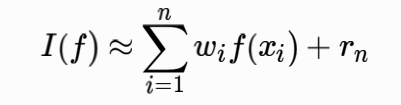其中rn可认为是偏差，一般可以忽略不计，wi可以视为权重。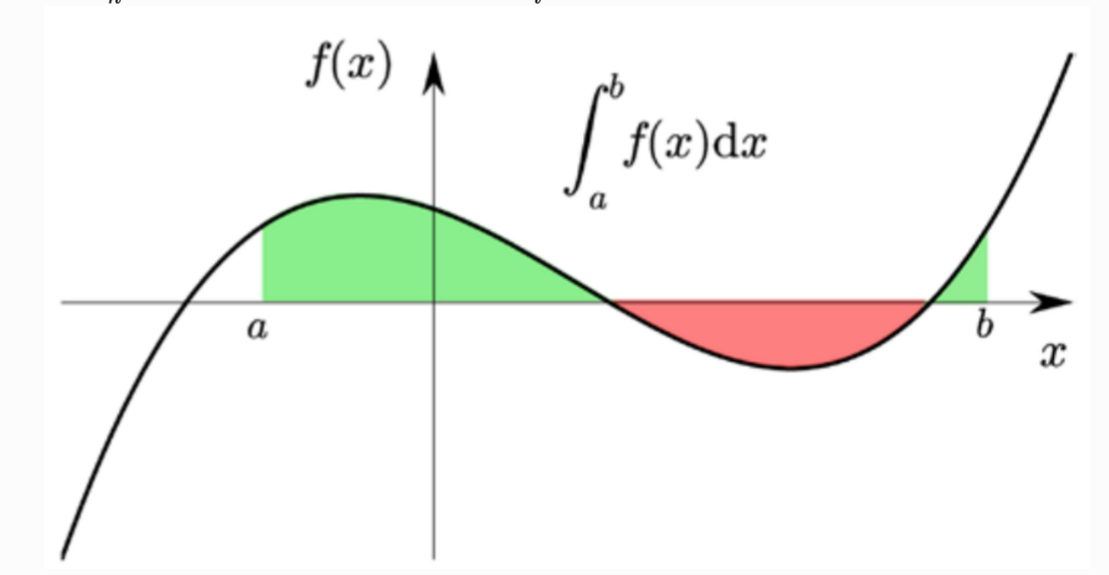在SciPy里提供了很多的求各类积分的函数，依据传入参数的不同可以分为两类：一类是传入一个已知的函数和积分的上下限;另一类是传入点集，这个适用于做完物理实现后收集的一些数据，但函数无法确定，但有很多的数据点，那么这些点包络下的面积是多少，也是积分问题，所以在SciPy里有针对点集求积分的函数，形式上函数的参数是数组或者列表。

1、已知函数型求积分

本节以几个问题的形式展示SciPy下如何求积分。

• 问题1：这里假设函数为f(x)=x+1,求积分的上下限为[1,2],数学表达式为：
•from scipy import integrate def f(x): return x + 1 v, err = integrate.quad(f, 1, 2) print v

程序的执行结果为：

2.5

from scipy import integrate
def f(x, a, b): return a * x + b v, err = integrate.quad(f, 1, 2, args = (-1, 1)) print v

程序的执行结果是：

-0.5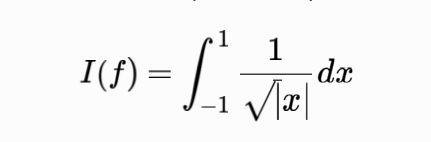from scipy import integrate
import numpy as np
def f(x): return 1 / np.sqrt(abs(x)) v, err = integrate.quad(f, -1, 1) print v

程序运行时：

scipy1801.py:4: RuntimeWarning: divide by zero encountered in double_scalars return 1 / np.sqrt(abs(x)) inf

结果是inf(无限、无穷)且有除0错误！ 修改一下：

from scipy import integrate
import numpy as np def f(x): return 1 / np.sqrt(abs(x)) v, err = integrate.quad(f, -1, 1, points=) print v

结果是：

4

我们可以绘制一下这个函数的可视化曲线：

from scipy import integrate
import numpy as np
def f(x): return 1 / np.sqrt(abs(x)) v, err = integrate.quad(f, -1, 1, points=) print v

import numpy as np, matplotlib.pyplot as plt
from mpl_toolkits.mplot3d.axes3d import Axes3D
fig, ax = plt.subplots(figsize=(8, 3))
x = np.linspace(-1, 1, 10000)
ax.plot(x, f(x), lw=2)
ax.fill_between(x, f(x), color=‘green’, alpha=0.5)
ax.set_xlabel(" x x ", fontsize=18)
ax.set_ylabel(" f ( x ) f(x) ", fontsize=18)
ax.set_ylim(0, 25)
plt.show()

得到如下的结果图：2 给出点集的积分

在无法确认积分函数的情况下，给出一些序列也可做积分。

from scipy import integrate import numpy as np def f(x): return np.sqrt(x) x = np.linspace(0, 2, 10) y = f(x) v = integrate.trapz(y, x) print v

程序的运行结果：

1.8652953655957172

3 多重积分

•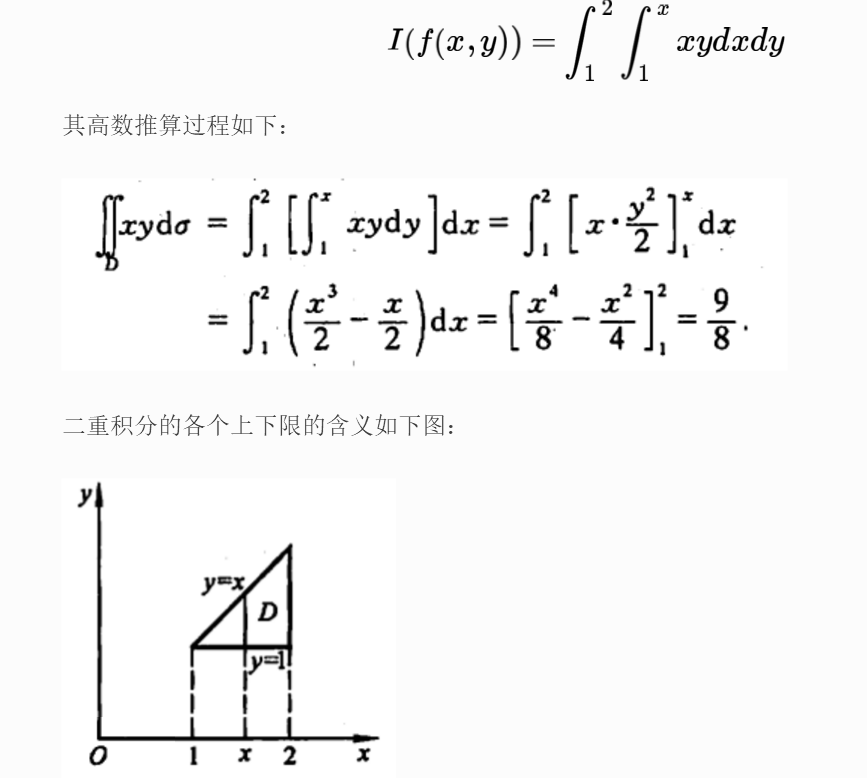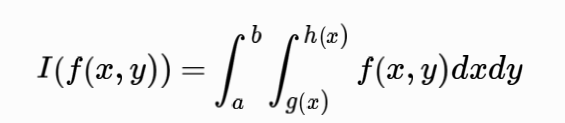from scipy import integrate import numpy as np def f(x, y): return x * y def h(x): return x v, err = integrate.dblquad(f, 1, 2, lambda x: 1, h) print v

程序的执行结果：

1.125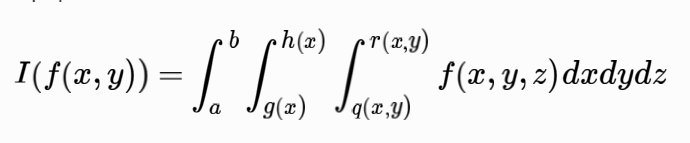tqlquad(f, a, b, g, h, q, r)

其中f、g、h、q、r均为函数。下面以计算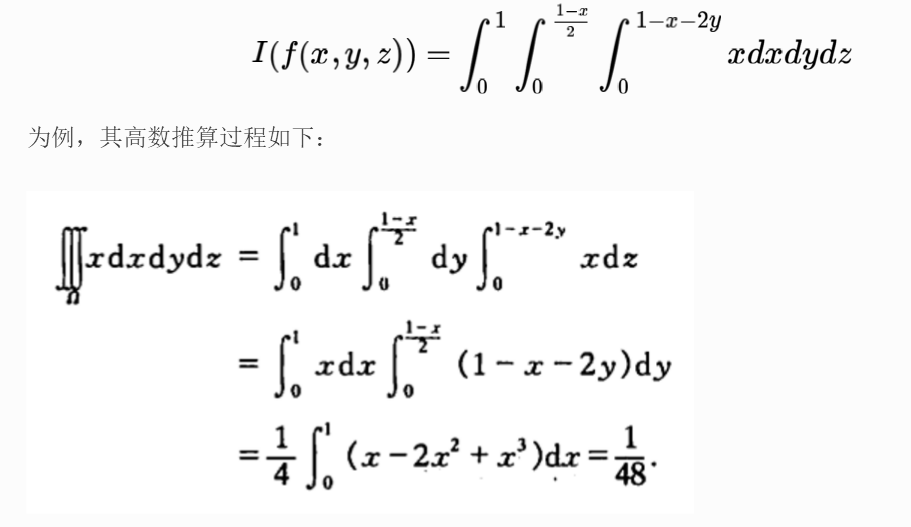用Python编写的程序如下所示：

from scipy import integrate import numpy as np f = lambda x, y, z : x g = lambda x : 0 h = lambda x : (1 - x) / 2 q = lambda x, y : 0 r = lambda x, y : 1 - x - 2 * y v, err = integrate.tplquad(f, 0, 1, g, h, q, r) print v

程序执行结果：

0.02083333333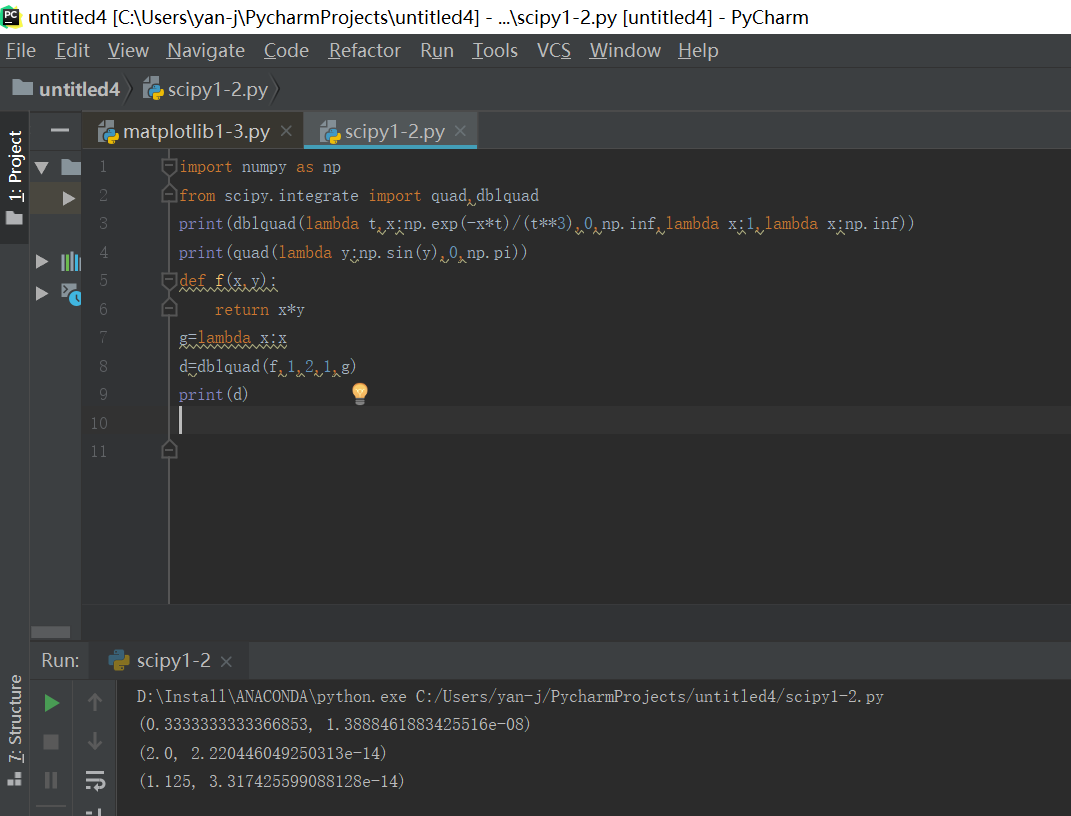[参考文献](https://www.cnblogs.com/Yanjy-OnlyOne/p/11185582.html)
展开全文旁白：当你创建一个符号表达式时，保持它的象征性。不要混合使用真正的浮点np.pi和复杂的浮点1j，而是使用SymPy的pi和{}。在from sympy import exp, pi, I, besselj, symbols

x, r = symbols('x r')

integrand = exp(-x**2) * exp(pi*I*(-x)) * besselj(0, r*x) * x

但是是的，它看起来并不能把贝塞尔函数的乘积与exp(-x**2) * exp(pi*I*(-x))积分。当r被1代替时，这种情况已经发生了，所以r的符号性质与此无关。在

直接回答您的问题：Is there any way of performing numerical integration with symbolic variables?

没有，就像没有干水一样。这在术语上是矛盾的。在Is the only other alternative defining the values of r a priori and finding the integral for each one of them?

是的。可以通过SymPy(称为mpmath)完成：

^{pr2}\$

考虑到这个函数的值很复杂，我们还不太清楚如何绘制这个函数。也许你是想画出强度的绝对值？在

from scipy.special import jn

integrand = lambda x, r: np.exp(-x**2) * np.exp(np.pi*1j*(-x)) * jn(0, r*x) * x

intensity = lambda r: np.sqrt(quad(lambda x: np.real(integrand(x, r)), 0, 5)**2 + quad(lambda x: np.imag(integrand(x, r)), 0, 5)**2)

现在，intensity(3)的计算速度比前一个版本快得多。我们可以把它画出来：import matplotlib.pyplot as plt

t = np.linspace(0, 3)

plt.plot(t, np.vectorize(intensity)(t))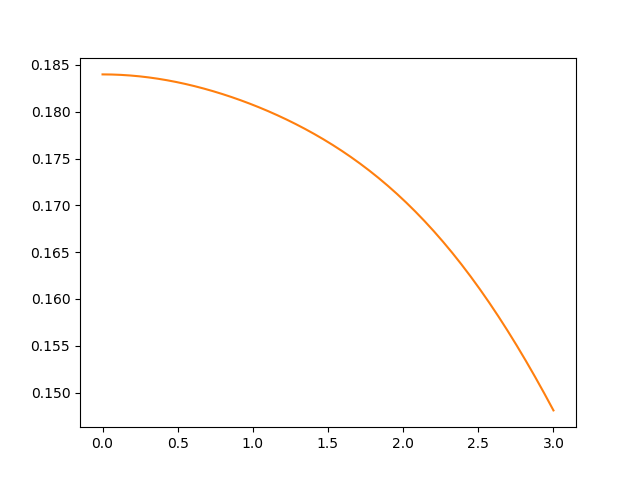展开全文• Python教你积分

2021-03-06 19:33:36
@Author：by Runsen原文：...通常分为定积分和不定积分两种。其实我也不会积分，水平太差了。 一重积分$$\int_1^2 x^2 dx$$用sympy计算from sympy import *x = symbols("x")print(int...
• 我需要解决的积分有以下形式：我必须为r的许多不同值求解这个积分,这既影响积分的极限,也影响积分(通过函数g).因此,我没有找到一种方法来对问题进行矢量化,而必须依赖于循环.这显着减慢了问题,因为我需要在每个循环...
• python SciPy包计算积分|Python技能树征题python 算法 scipy
• 文章目录积分图原理效果演示 积分图原理 关于积分图原理：https://www.cnblogs.com/magic-428/p/9149868.html 第一个提出 Haar 特征快速计算方法的是 CVPR2001上 的那篇经典论文 [《Rapid object detection using a ...opencv 计算机视觉 python
• #coding:utf-8'''微积分'''import sympysympy.init_printing()from sympy import I, pi, ooimport numpy as np# 1、求函数的导数x = sympy.Symbol('x')y = sympy.Symbol('y')z = sympy.Symbol('z')f = sympy....
• 我有点困在一个函数上，我试图通过scipy，python进行数值积分。在为了简单起见，我将函数定义为：integral f(x,y)= SUM[double integral(ax+by)dxdy]a和b是常数，但对于每个积分的方程，它们是不同的。我已经将每个...
• # Do in Python # # >>> import pyximport; pyximport.install(reload_support=True) # >>> import cythonmodule cimport numpy as np cimport cython cdef extern from "complex.h": double complex csqrt(double ...
• 谢谢之路。我去知乎，和百度搜了一圈。很多都说 sage 在符号运算方面比肩 mathematica 。开源免费。...形成这种印象的原因是：在知乎搜索 python 积分 出来的大部分都介绍 sympy 的方法。在知乎搜索“...
• 编译环境：ubuntu17.04 Python3.5所需库：numpy、scipy、matplotlib下面是理想平面的辐射强度计算(课程大作业～～～)1、超越函数积分运算def integral(x,c1,c2,T):return ((c1*0.98)/(x**5))*(1/((np.e**(c2/(x*T)))...
• I am trying to solve the following equation in python using the scipy.odeint function.Currently I am able to implement this form of the equationin python using the following script:def dY(y1, x):a = 0...
• 积分是一种非常重要的“数学分析”思想(方法)，在许多领域中都有应用，比如：计算平面面积、曲线长度、空间图形的体积、旋转曲面面积和物理学中的“微元法”等。而如何用好“微积分”是这部分学习的重点。要用好微...
• \n积分:完成学校所给的任务后所得积分累加值,可兑换奖励。\n星级：积分值越大，星级越高。\n称号：完成学校的某项特殊任务时获得，拥有称号的学生对应的成长值就高。\n成长值:学生每天积分会自动按成长值增加。" ...python
• 继续承接上文学习Scipy科学数据处理，为我们后面Python大数据处理开发打基础。今天主要学习分享的是数值积分。对于积分，相信大家都不陌生，上过中学的都有学过。最常见的是利用定积分求曲线面积等等。定积分最熟悉...
• 示例已知积分公式如下求[0.5，5]上积分，即求下图阴影部分面积根据积分公式求源函数等于：则确切解等与F(5)-F(0.5)=3.9002072872864524当不知道源函数时使用以下方法可以求得积分面积首先定义函数def func(x):return...
• from scipy.special import roots_legendre ...a,b = 0,2 #积分上下限 x,w = roots_legendre(N) xp = x*(b-a)/2+(b+a)/2 wp = w*(b-a)/2 s = 0 for i in range(N): s+=wp[i]*f(xp[i]) print(s)
• print('-'*25,'我的计算器','-'*25)def calculator():result = 0while True:try:s = input("请输入数字[到0结束]:")if s == "0":print("重置输入N,退出输入Y!")confirm = input('请选择[Y/N]:')if confirm in "Y, y...
• 积分有三种形式，并且都用的是integrate（）方法 求不定积分：integrate(expr, var) 求定积分：integrate(expr, (var, min, max)) 求多重积分：integrate(expr, (var1, min, max),(var2,min,max)) （2）源代码： ...python微积分库
• 我迫切需要加快一些代码,包括在两个步骤1d插值二重积分。我将其简化为以下最小工作示例:import numpy as npfrom scipy.interpolate import interp1d as interpfrom scipy.integrate import rombergdef calculate_p22...
• 根据维基百科。...Ei(x)=−∫−x∞e−ttdt=∫−∞xettdtE_i\left ( x \right )=-\int_{-x}^{\infty}\frac{e^{-t}}{t}\mathrm{d}t=\int_{-\infty}^x\...接下来，我们计算−Ei(−x)-E_i\left(-x\right)−Ei​(−x)的积分
• Python求定积分+处理can't convert expression to float错误python 定积分
• python符号积分

2021-06-20 13:25:18
积分和求导的关系如下： 符号运算可以用sympy模块完成。 先导入init_printing模块方便其显示
• 本文内容来自学习麻省理工学院公开课：单变量微积分-隐函数微分法和逆函数导数-网易公开课 和麻省理工学院公开课：单变量微积分习题课-除法法则-网易公开课 一、反函数 1、定义 通常 这些就是反函数了 ...python 机器学习 数据挖掘 数学建模
• 求f(x) = sin(x)/x 的不定积分和负无穷到正无穷的定积分sin(x)/x 的不定积分是信号函数sig ，负无穷到正无穷的定积分为piimport mathimport numpy as npimport matplotlib.pyplot as pltfrom sympy import * #用于...
• 线性回归模型是最简单的机器学习模型，基础可以从线性回归模型开始入手，慢慢地过渡到非线性回归以及神经网络模型。1.概念在统计学中，线性回归(Linear Regression)是利用称为线性回归方程的最小平方函数对一个或多......

python积分python 订阅